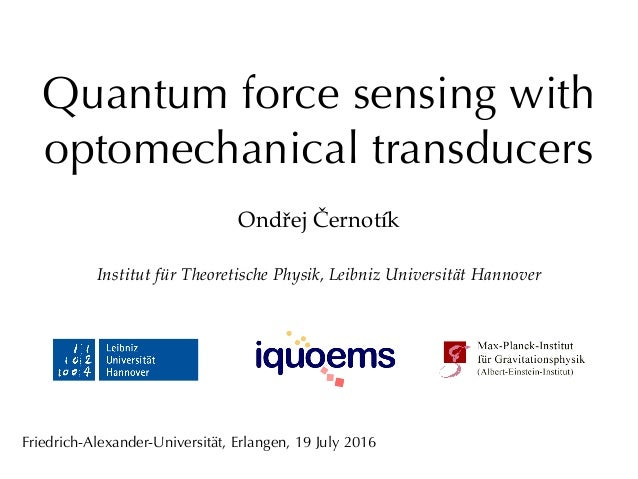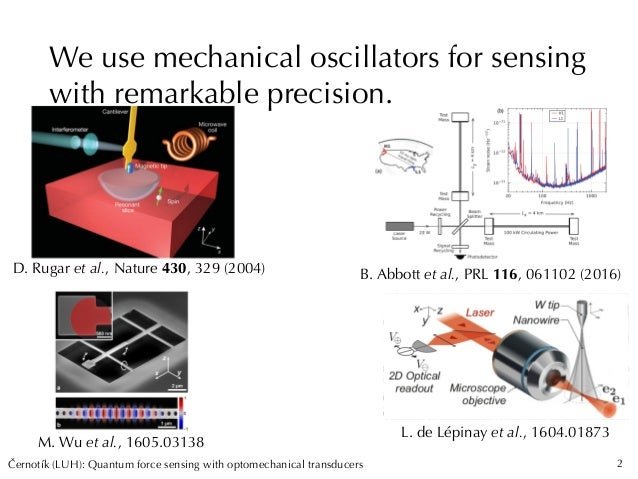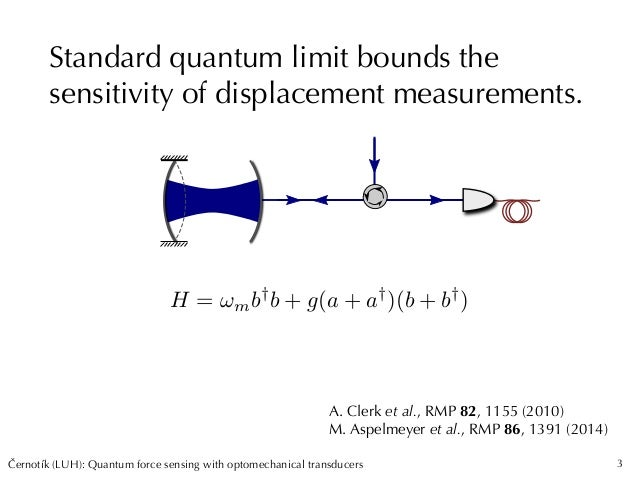Successfully reported this slideshow.Upcoming SlideShare
×

ofUpcoming SlideShare
Next

1 Like

Share

# Quantum force sensing with optomechanical transducers

Optomechanical force sensing is an established measurement technique that can reach remarkable precision. In most applications, the system exerting the force on the mechanical oscillator is treated classically and we are not interested in any coherence between states of the system that give rise to different forces. A full quantum treatment, however, enables richer physics since measuring more such systems can lead to interference effects.
In this talk, I will show that the coherence can survive the measurement and can be used for quantum-technological applications. I will consider a model example of spin readout in superconducting qubits. Coupling two transmon qubits to mechanical oscillators and reading out the mechanical positions using a single beam of light provides information on the total spin of the qubits. It is thus possible to conditionally generate entanglement between the two qubits. The system represents a basic quantum network with superconducting circuits. The scheme has modest requirements on the system parameters; it does not require ground-state cooling or resolved-sideband regime and can work with quantum cooperativity moderately larger than unity.
Afterwards, I will consider another scheme, namely nondestructive detection of a single photon using an optomechanical transducer. The basic idea is similar to spin readout; the photon exerts a force on a mechanical oscillator and the the force is measured optically. I will argue that such a measurement is subject to a quantum limit due to backaction of the transducer on the dynamics of the photon and that this result also applies to other techniques of nondestructive photon detection, such as methods using Kerr interaction between the single photon and a meter beam. Finally, I will show numerically that measurement backaction can be evaded when the measurement rate is suitably modulated.

See all

See all

### Quantum force sensing with optomechanical transducers

1. 1. Quantum force sensing with optomechanical transducers Ondřej Černotík Institut für Theoretische Physik, Leibniz Universität Hannover Friedrich-Alexander-Universität, Erlangen, 19 July 2016
2. 2. Cernotík (LUH): Quantum force sensing with optomechanical transducersˇ D. Rugar et al., Nature 430, 329 (2004) B. Abbott et al., PRL 116, 061102 (2016) M. Wu et al., 1605.03138 L. de Lépinay et al., 1604.01873 We use mechanical oscillators for sensing with remarkable precision. 2
3. 3. Cernotík (LUH): Quantum force sensing with optomechanical transducersˇ Standard quantum limit bounds the sensitivity of displacement measurements. 3 A. Clerk et al., RMP 82, 1155 (2010) M. Aspelmeyer et al., RMP 86, 1391 (2014) H = !mb† b + g(a + a† )(b + b† )
4. 4. Cernotík (LUH): Quantum force sensing with optomechanical transducersˇ Standard quantum limit bounds the sensitivity of displacement measurements. 4 A. Clerk et al., RMP 82, 1155 (2010) M. Aspelmeyer et al., RMP 86, 1391 (2014) S2 imp =  16g2 ✓ 1 + 4 !2 2 ◆ m(!) = [m(!m !)2 im !] 1 S2 ba = 4~2 g2  ✓ 1 + 4 !2 2 ◆ 1 | m(!)|2
5. 5. Cernotík (LUH): Quantum force sensing with optomechanical transducersˇ I will talk about: 5 - OC and K. Hammerer, PRA (accepted)ˇ Entanglement of superconducting qubits Optomechanical single-photon detection
6. 6. Cernotík (LUH): Quantum force sensing with optomechanical transducersˇ Entanglement of superconducting qubits 6 - OC, K. Hammerer, PRA (accepted)ˇ
7. 7. Cernotík (LUH): Quantum force sensing with optomechanical transducersˇ Superconducting systems are among the best candidates for quantum computers. 7 Schoelkopf • Controlling microwave ﬁelds with qubits Hofheinz et al., Nature 454, 310 (2008); Nature 459, 546 (2009) • Feedback control of qubits Ristè et al., PRL 109, 240502 (2012); Vijay et al., Nature 490, 77 (2012); de Lange et al., PRL 112, 080501 (2014) • Entanglement generation Ristè et al., Nature 502, 350 (2013); Roch et al., PRL 112, 170501 (2014); Saira et al., PRL 112, 070502 (2014) • Quantum error correction Córcoles et al., Nature Commun. 6, 6979 (2015), Kelly et al., Nature 519, 66 (2015), Ristè et al., Nature Commun. 6, 6983 (2015)
8. 8. Cernotík (LUH): Quantum force sensing with optomechanical transducersˇ We can generate entanglement by measurement and postselection. 8 C. Hutchison et al., Canadian J. Phys. 87, 225 (2009) N. Roch et al., PRL 112, 170501 (2014) Hint = za† aDispersive coupling |11i |00i |01i + |10i
9. 9. Cernotík (LUH): Quantum force sensing with optomechanical transducersˇ We want to extend the distance over which the qubits become entangled. 9 - Other proposals: K. Stannigel et al., PRL 105, 220501 (2010) B. Clader, PRA 90, 012324 (2014) Z. Yin et al., PRA 91, 012333 (2015) Experiments: J. Bochmann et al., Nat. Physics 9, 712 (2013) R. Andrews et al., Nat. Physics 10, 321 (2014) T. Bagci et al., Nature 507, 81 (2014) K. Balram et al., Nat. Photon. 10, 346 (2016)
10. 10. Cernotík (LUH): Quantum force sensing with optomechanical transducersˇ Optomechanical transducer acts as a force sensor. 10 F = ~ /( p 2xzpf ) S2 F (!) = x2 zpf /[8g2 2 m(!)]Sensitivity: ! ⌧ !m ⌧meas = S2 F (!) F2 = !2 m 16 2g2 ⌧ T1,2Measurement time: H = z(b + b† ) + !mb† b + g(a + a† )(b + b† )
11. 11. Cernotík (LUH): Quantum force sensing with optomechanical transducersˇ The thermal mechanical bath affects the qubit. 11 mech = S2 f (!) = 2 2 !2 m ¯nDephasing rate: ⌧meas < 1 mech ! C = 4g2  ¯n > 1 2
12. 12. Cernotík (LUH): Quantum force sensing with optomechanical transducersˇ The system can be modelled using a conditional master equation. 12 D[O]⇢ = O⇢O† 1 2 (O† O⇢ + ⇢O† O) H[O]⇢ = (O hOi)⇢ + ⇢(O† hO† i) H. Wiseman & G. Milburn, Quantum measurement and control (Cambridge) d⇢ = i[H, ⇢]dt + Lq⇢dt + 2X j=1 {(¯n + 1)D[bj] + ¯nD[b† j]}⇢dt + D[a1 a2]⇢dt + p H[i(a1 a2)]⇢dW H = 2X j=1 j z(bj + b† j) + !mb† jbj + g(aj + a† j)(bj + b† j) + i  2 (a1a† 2 a2a† 1)
13. 13. Cernotík (LUH): Quantum force sensing with optomechanical transducersˇ The transducer is Gaussian and can be adiabatically eliminated. 13 OC et al., PRA 92, 012124 (2015)ˇ 2 qubits Mechanics, light
14. 14. Cernotík (LUH): Quantum force sensing with optomechanical transducersˇ We obtain an effective equation for the qubits. 14 d⇢q = 2X j=1  1 T1 D[ j ] + ✓ 1 T2 + mech ◆ D[ j z] ⇢qdt + measD[ 1 z + 2 z]⇢qdt + p measH[ 1 z + 2 z]⇢qdW meas = 16 2 g2 !2 m , mech = 2 !2 m (2¯n + 1)
15. 15. Cernotík (LUH): Quantum force sensing with optomechanical transducersˇ Optical losses introduce additional dephasing. 15 (1 ⌧) measD[ 1 z]⇢q p ⌘ measH[ 1 z + 2 z]⇢q
16. 16. Cernotík (LUH): Quantum force sensing with optomechanical transducersˇ A transmon qubit can capacitively couple to a nanobeam oscillator. 16 = 2⇡ ⇥ 5.8 MHz g = 2⇡ ⇥ 900 kHz  = 2⇡ ⇥ 39MHz !m = 2⇡ ⇥ 8.7 MHz Qm = 5 ⇥ 104 T = 20 mK ¯n = 48 T1,2 = 20 µs C = 10 ⌘ Psucc Psucc OC and K. Hammerer, PRA (accepted), 1512.00768ˇ
17. 17. Cernotík (LUH): Quantum force sensing with optomechanical transducersˇ Optomechanical single-photon detection 17
18. 18. Cernotík (LUH): Quantum force sensing with optomechanical transducersˇ We measure the force with which a photon kicks the oscillator. 18 Hint = g0a† 2a2(b + b† ) a1 a2 d⇢ =  2 [a† 1a2 a† 2a1, ⇢]dt + D[a1 + a2]⇢dt + D[a† 2a2]⇢dt + p ⌘ H[a† 2a2]⇢dW
19. 19. Cernotík (LUH): Quantum force sensing with optomechanical transducersˇ The measurement results in quantum Zeno effect. 19 Coupling Measurement MeasurementS0 = 1 2 (a† 1a1 + a† 2a2) S1 = 1 2 (a† 1a2 + a† 2a1) ⇠ x S2 = 1 2i (a† 1a2 a† 2a1) ⇠ y S3 = 1 2 (a† 1a1 a† 2a2) ⇠ z J. Gambetta et al., PRA 77, 012112 (2008) [Si, Sj] = i"ijkSk [S0, Si] = 0
20. 20. Cernotík (LUH): Quantum force sensing with optomechanical transducersˇ We estimate the error by calculating the (Gaussian) signal-to-noise ratio. 20 ˙⇢ =  2 [a† 1a2 a† 2a1, ⇢] + D[a1 + a2]⇢ + D[a† 2a2]⇢ J(T) = Z T 0 dt S(t)I(t) I(t), S(t) / n1(t) SNR = s Z T 0 dt n2 1(t) + p H[a† 2a2]⇢dW/dt Idt = 2 p ha† 2a2idt + dW Dark counts Missed photons Average error
21. 21. Cernotík (LUH): Quantum force sensing with optomechanical transducersˇ Modulated measurement We can partially avoid the measurement backaction. 21 Off–on measurement Modulated measurement (t) = sin(!t) (t) = ⇥(t t0)
22. 22. Cernotík (LUH): Quantum force sensing with optomechanical transducersˇ We also need better control over the photon to improve incoupling. 22
23. 23. Cernotík (LUH): Quantum force sensing with optomechanical transducersˇ • Strong optomechanical cooperativity, • Sufﬁcient qubit lifetime I talked about: 23 - OC and K. Hammerer, PRA (accepted)ˇ Entanglement of superconducting qubits C = 4g2  ¯n > 1 2
24. 24. Cernotík (LUH): Quantum force sensing with optomechanical transducersˇ I talked about: 24 Optomechanical single-photon detection • Quantum Zeno effect limits the efﬁciency • We need backaction evasion and better incoupling
•#### MohamedHamdy342

Nov. 27, 2019

Total views

67

On Slideshare

0

From embeds

0

Number of embeds

0

1

Shares

0